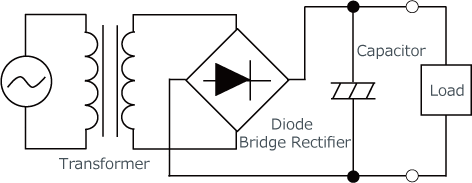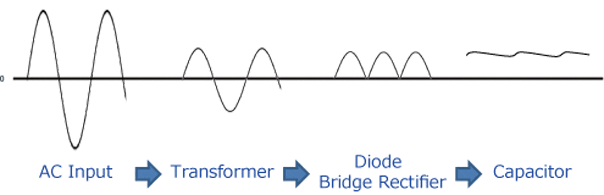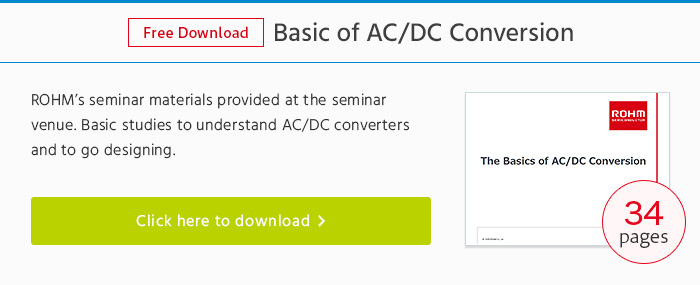# Transformer Method (AC-DC Converter)

## AC-DC Conversion Method

There is a transformer method and switching method for AC-DC conversion.
Here we will cover the Transformer method.

### Transformer Method

Shown here is the transformer circuit configuration of a typical AC-DC converter.

【Transformer-Type Circuit Configuration Example】The figure below shows the transition of the voltage waveform using the transformer method.
In the transformer method, the input AC voltage is first stepped down to an appropriate level using a transformer (e.g. 100VAC to 10VAC). This is AC-AC conversion, and the step-down voltage is set via the winding ratio of the transformer.
Next, the stepped down AC voltage is full-wave rectified using a diode bridge rectifier and converted into pulsed DC.
Finally, a capacitor is used to smooth the waveform, resulting in DC output with small ripple voltage. This is the most common way of performing AC-DC conversion.

【Waveform Transition (Transformer Method)】